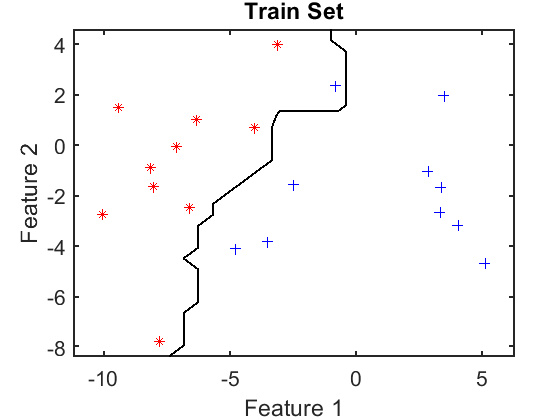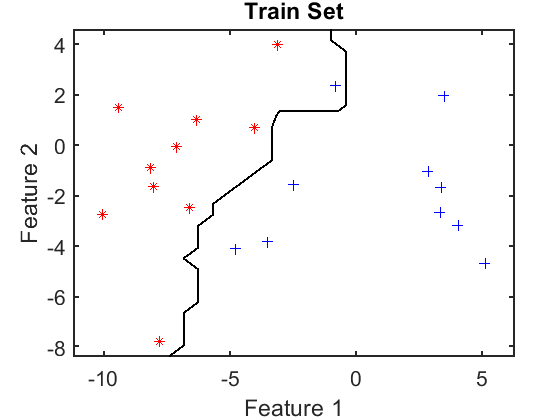# classifiers

Introduction of defining, training and evaluating classifiers

PRTools and PRDataFiles should be in the path

Download the m-file from here. See http://37steps.com/prtools for more.

## Contents

```prwaitbar off                % waitbar not needed here
delfigs                      % delete existing figures
randreset(1);                % takes care of reproducability
```

## Define a classifier

```u = knnc([],3);              % the untrained 3-NN classifier
```

## Define datasets for training and testing

```a = gendatb([20 20],2);      % define dataset
a = setlablist(a,[' A ';' B ']); % define class names
[t,s] = gendat(a,0.5);       % split it 50-50 in train set and test set
t = setname(t,'Train Set');  % name the train set
s = setname(s,'Test Set');   % name the test set
```

## Train the classifier

```w = t*u;                     % train the classifier
```

## Show the trained classifier on the training set

```figure;
scatterd(t);                 % show training set
axis equal
plotc(w);                    % plot classifier
V = axis;

dt = t*w;                    % apply classifier to the training set
et = dt*testc;               % compute its classification error
fprintf('The apparent error: %4.2f \n',et); % print it
labt = getlabels(t);         % true labels of training set
labtc= dt*labeld;            % estimated labels of classified training set
disp([labt labtc]);          % show them. They correspond to the estimated error
```
```The apparent error: 0.05
A  A
A  B
A  A
A  A
A  A
A  A
A  A
A  A
A  A
A  A
B  B
B  B
B  B
B  B
B  B
B  B
B  B
B  B
B  B
B  B
```Compute the apparent error and show the estimated and true labels in classifying the training set. They corespond to the apparent error and the classifier in the scatter plot

## Show the trained classifier on the test set

```figure;
scatterd(s);                 % show test set
axis(V);
plotc(w);                    % plot classifier

ds = s*w;                    % apply classifier on the test set
es = ds*testc;               % compute its classification error
fprintf('The test error: %4.2f \n',es); % print it
labs = getlabels(t);         % true labels of test set
labsc= ds*labeld;            % estimated labels of classified test set
disp([labs labsc]);          % show them. They correspond to the estimated error
```
```The test error: 0.20
A  A
A  A
A  A
A  A
A  B
A  A
A  A
A  A
A  A
A  A
B  B
B  A
B  B
B  B
B  B
B  B
B  B
B  A
B  B
B  A
```Compute the test error and show the estimated and true labels in classifying the test set. They corespond to the test error and the classifier in the scatter plot